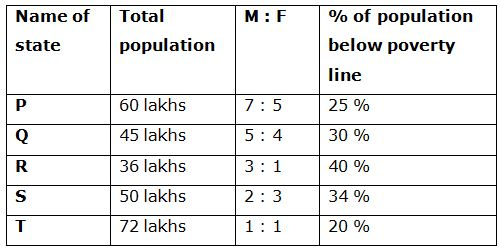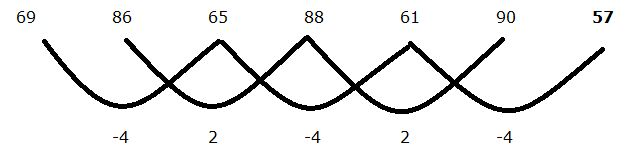# gkrecall

We brings you daily Current affairs, daily Current Affairs Quiz, weekly Current Affairs, weekly Current Affairs Quiz to enhance your preparation for upcoming exams. We also provide articles related to quant, reasoning, English, ssc etc .

## QUANT PRACTICE SET 7 FOR RRB PO & CLERK PRELIM 2020

RRB Clerk and RRB PO Prelims exam are going to be held in the upcoming months. We have already provided you with the PDFs of many topics of Quantitative Aptitude like Simplification/Approximation, Number Series,datainterpretation.important Arithmetic questions to prepare for RRB Clerk and PO Pre exam. Practicing these questions will help you to know about the level of the questions. To increase your speed and accuracy, enhance your calculations.

### START QUIZ

Directions (01 – 05): Find out the wrong number in the following number series.
1) 19, 235, 260, 324, 335, 341
a) 341
b) 235
c) 260
d) 335
e) 324
2) 69, 86, 65, 88, 61, 90, 56
a) 86
b) 61
c) 56
d) 88
e) 90
3) 13, 94, 45, 70, 60, 62
a) 94
b) 60
c) 70
d) 62
e) 45
4) 23, 46, 92, 161, 252, 268
a) 46
b) 161
c) 252
d) 92
e) 268
5) 27, 54, 162, 448, 3240, 19440
a) 54
b) 3240
c) 162
d) 448
e) 19440
Directions (Q. 6 – 10): Study the following information carefully and answer the given questions:
The following table shows the total population in 5 different states, the ratio of male and female among them and the percentage of population below poverty line.6) Find the difference between the total male population in state P and R together to that of total female population in state Q and T together?
a) 15 lakhs
b) 22 lakhs
c) 6 lakhs
d) 10 lakhs
e) None of these
7) Find the ratio between the above poverty line population in state R to that of the below poverty line population in state S?
a) 108: 85
b) 75: 62
c) 52: 43
d) 11: 5
e) None of these
8) Find the total number of female population in state P, R and S together
a) 72 lakhs
b) 90 lakhs
c) 56 lakhs
d) 64 lakhs
e) None of these
9) The above poverty line population in state P and Q together is approximately what percentage of total population in state S?
a) 132 %
b) 153 %
c) 120 %
d) 105 %
e) 94 %
10) The below poverty line population in state R and T together is what percentage more/less than the total male population in state Q and S together?
a) 36 % more
b) 15 % less
c) 36 % less
d) 4 % more
e) 15 % more
SOLUTIONS

19 + 63 = 235
235 + 52 = 260
260 + 43 = 324
324 + 32 = 333
333 + 23 = 341
The wrong number is 335.
69             86             65             88             61             90             57
17             -21            23             -27            29             -33
4              2              4              2              4
Or,The wrong term is 56.
13 + 92 = 94
94 – 72 = 45
45 + 52 = 70
70 – 32 = 61
61 + 12 = 62
The wrong term is 60
23 + 23 * 1 = 46
46 + 23 * 2 = 92
92 + 23 * 3 = 161
161 + 23 * 4 = 253
253 + (23 * 5) = 368
The wrong term is 252.
27 * 1 = 27
27 * 2 = 54
54 * 3 = 162
162 * 4 = 648
648 * 5 = 3240
3240 * 6 = 19440
The wrong term is 448
Direction (6-10) :
The total male population in state P and R together
= > 60*(7/12) + 36*(3/4)
= > 35 + 27 = 62 lakhs
The total female population in state Q and T together
= > 45*(4/9) + 72*(1/2)
= > 20 + 36 = 56 lakhs
Required difference = 62 – 56 = 6 lakhs
The above poverty line population in state R
= > 36*(60/100)
The below poverty line population in state S
= > 50*(34/100)
Required ratio = [36*(60/100)]: [50*(34/100)]
= > 108: 85
The total number of female population in state P, R and S together
= > 60*(5/12) + 36*(1/4) + 50*(3/5)
= > 25 + 9 + 30 = 64 lakhs